Next: The logical operations Up: Alan Turing's Manual for Previous: Examples of programmes on

# The multiplier and the double length accumulator

We shall now begin to describe the various respects in which the full machine differs from the reduced machine. Most of these differences are essentially independent. One may satisfactorily learn the effect of each difference as if added to the reduced machine in the absence of the others, and having learnt these effects will be in a position to manage the whole machine.

We begin by considering the effect of adding a multiplier to the machine. If we regard a standard number as consisting of forty binary digits then the product of two such numbers will occupy eighty digits. For this reason it is necessary to have an eighty digit accumulator. This decision in itself requires us to adopt a more sophisticated attitude to our numbers and our rows of digits. In the reduced machine it was almost possible to regard the long lines as representing integers, but it was necessary to admit that they were really to be reckoned modulo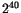. Long lines with a 1' in the most significant place could be regarded as representing negative numbers, provided that it is accepted that positive numbers greater thancannot be represented. With the double length accumulator similar considerations apply with greater complexity. Numbers held in the store must be reckoned moduloas before, but numbers in the accumulator must be reckoned modulo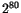.

In order to be able to express these matters clearly it is necessary to have notations which draw the essential distinctions, though these distinctions may appear pedantic, and though in a majority of applications the notation is not needed in all its detail. We distinguish therefore between rows of digits' and numbers. I do not think that either of these expressions needs much elaboration. By numbers I shall mean real numbers. The content of any part of the machine will be a row of digits or an assembly of such rows, and not a number (with the exception of the multiplicand). Additions and multiplications are however performed on numbers and not rows of digits. However, in order that the processes of the machine may be described in terms of these operations it is necessary to be able to relate rows of digits to numbers, and vice-versa.

It would be sufficient in theory to be able to connect one number with each row, in such a way that all rows got different numbers. In practice four possible conventions present themselves particularly forcibly. We assume that our row of digits is of length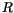and the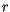th digit is.

• The plus-convention: The associated number is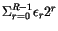• The plus-or-minus convention: The associated number is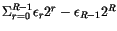• The fractional plus-convention: The associated number is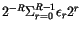• The fractional plus-or-minus convention: The associated number is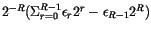We shall use all of these conventions, both in connection with the store lines, and with the accumulator. To convert a row into a number according to one of these conventions one writes respectively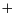,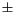,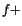, or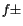as a suffix after the content of the row.

As regards the converse process, that of defining rows of digits in terms of numbers it will suffice to be able to take any sequence of consecutive digits from the binary expansion of a number. Accordingly we say that for any real number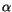, and integers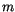,for which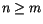,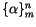is the row of digits forming the coefficients of theth to theth powers of two in the binary expansion of. If possible this expansion is to be terminating.

A number of statements are made below in illustration of these conventions.

1.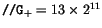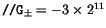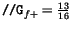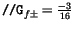2.provided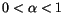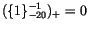3.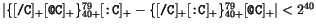A further convention which may be used in this connection is the use of the symbol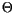. This is somewhat analogous to the use of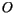,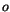in analysis. One uses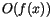to mean some function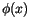such that there exists a positive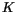satisfying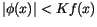for all sufficiently large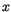'. There is also an understanding that the functions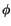and constantsmay be different at every appearance of. The use ofis similar.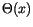means simply some quantitysatisfying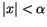' and again may be different on every appearance. Thus for instance from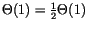one cannot conclude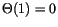for the two appearances of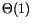might have the values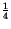and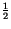. Using this convention 2) above could be written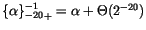providedIn terms of these conventions we may explain the properties of the multiplier as follows. To describe the state of the Mark II machine we give the states of the stores and control as in the reduced machine, but the accumulator is an eighty digit one. We also have to describe the state of the multiplicand'. This is considered to be an integer which may take any value from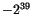to. In this respect the multiplicand is exceptional. The contents of all other parts of the machine are considered as rows of digits. Having explained this a number of further function symbols should become intelligible, viz. those marked m in the list below.

[The appendices to the manual do contain a list of instructions (in fact, two; one as part of a quick-reference sheet), but oddly, neither is marked as described. From those lists, the function symbols directly relevant to the multiplier are as follows, where D denotes the value of the multiplier:

 Function symbol Equations /C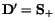/K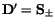/¼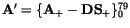/D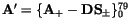/N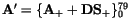/F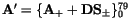There are several additional function symbols whose properties can only be explained (or must be redefined) with reference to the 80-bit accumulator of the real machine, as opposed to the 40-bit accumulator of Turing's `reduced machine''. If we define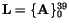and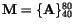, then these are as follows:
 Function symbol Equations /E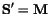/A,/S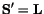/I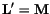,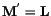/U,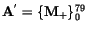T/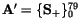TA,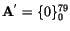T: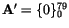TI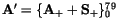T¼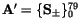TN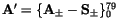TF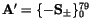TC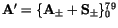TK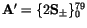Further supplementary tables of function codes will be added after subsequent sections, except where the relevant instructions are specifically described in the original text.]

We may allow certain abbreviations as admissible, viz.

(a) Where it is clear that a row of digits is meant, and the number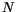of these digits is known one may write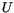for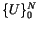, whereis an expression representing a real number, e.g., the equation for /F may be abbreviated fromto.

(b) When it is evident that a real number is meant, and it is irrelevant whether a suffixoris used (or irrelevant whetheroris used) one may omit the suffix (or use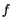only) e.g. the equation for /F may be abbreviated further to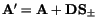.

[Turing makes little use of the notation introduced here in the rest of the manual, except briefly towards the end when discussing systematic errors in programs.]Next: The logical operations Up: Alan Turing's Manual for Previous: Examples of programmes on
Robert S. Thau 2000-02-13